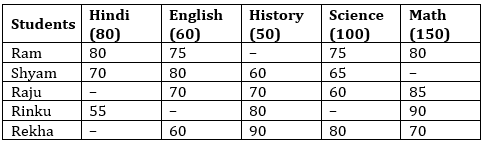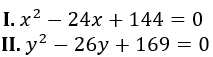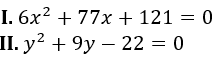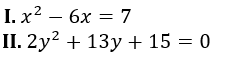Dear Students,

### Quantitative Aptitude Quiz for IBPS Clerk

Numerical Ability or Quantitative Aptitude Section has given heebie-jeebies to the aspirants when they appear for a banking examination. As the level of every other section is only getting complex and convoluted, there is no doubt that this section, too, makes your blood run cold. The questions asked in this section are calculative and very time-consuming. But once dealt with proper strategy, speed, and accuracy, this section can get you the maximum marks in the examination. Following is the Quantitative Aptitude quiz to help you practice with the best of latest pattern questions.

Directions (1-5): In following tabular chart, the percentage of marks in five different subjects obtained by 5 different students is given with their maximum marks in each subject respectively. Study the chart carefully and answer the questions that follows.Note: Some data are missing. If require then find them first and then solve the given questions.

Q1. If average mark obtained by all the five students in English is 70 percent, then find the missing data in English

70%
65%
75%
80%
60%

Q2. If marks obtained by Ram in History is 20% less than the mark obtained by Shyam in the same subject, then find the mark obtained by Ram in History
25
35
30
28
24

Q3. If Rekha obtained 10% less mark in Hindi than that of Shyam in the same subject, then find the average mark obtained by Rekha in all subjects(approximately)
57
63
71
75
52

Q4. If total marks obtained by Rinku in all the five subjects is 338 then find the mark obtained by Rinku in Science. (Use data for English obtained in Q1 to solve this question)
75
80
85
70
None of these

Q5. What is the marks obtained by Shyam in Math if marks obtained by Ram in Science is 20% less than that of marks obtained by Shyam in math?
93.75
95.7
105
102.75
None of these

Q6. A bag contains 4 black, 3 white and 8 blue color balls. If 4 balls are taken at random then the probability that all four balls are blue color ?
3/13
2/39
2/13
4/39
5/13

Q7. Three cards are drawn from pack of 52 cards. What is the probability that all are queens: (if replacement is required)
1/1331
1/2197
1/456
5/1331
3/5

Q8. 7 people are to be seated in a circular table. If Ravi and Shyam do not sit with each other. how many possible ways are there to sit around the circular table ?
462
720
120
240
480
Solution:
Number of possible ways when Ravi and Shyam sit with each other in circular table = 5! × 2
Total number of ways to sit in circular table = (7 – 1)! = 6!
Total number of ways when Ravi and Shyam do not sit with each other in circular table =
6! – 5! × 2
= 720 – 240
= 480

Q9. How many different words can be formed using the letters of the “QUESTION” such that words starts with T and ends with vowels ?
2808
8208
7204
2880
2472
Solution:
Word starts with T so one place is fixed and there are 6 places are keep changing and last place is for vowels
Total number of words = 1 × 6! × 4
= 720 × 4
= 2880

Q10. A wheel can cover 132 km in 3000 rotation. Find the radius of wheel ?
7 meters
21 meters
3.5 meters
15 meters
28 meters

Q11.if x < y
if x > y
if x = y
if x ≥ y
if x ≤ y or no relationship can be established between x and y.

Q12.if x < y
if x > y
if x = y
if x ≥ y
if x ≤ y or no relationship can be established between x and y.

Q13.if x < y
if x > y
if x = y
if x ≥ y
if x ≤ y or no relationship can be established between x and y.

Q14.if x < y
if x > y
if x = y
if x ≥ y
if x ≤ y or no relationship can be established between x and y.

Q15.if x < y
if x > y
if x = y
if x ≥ y
if x ≤ y or no relationship can be established between x and y.

You May also like to Read: